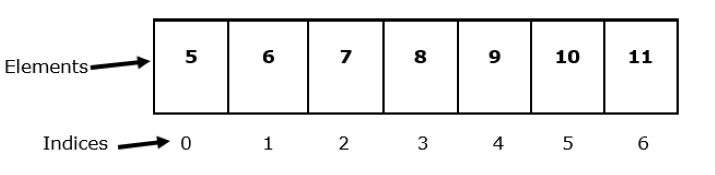# Java Program for Minimum product subset of an array

Array is a linear data structure that is used to store a group of elements with similar datatypes. It stores data in a sequential manner. Once we create an array we can’t change its size i.e. it is of fixed length.

The problem statement states that for a given array we have to find the minimum product of its subset. In this article, we will try to find the solution to the given problem.

## Program for minimum product of subsets

### Example 1

Let’s try to understand the problem and possible solution through an example.For the above array a few possible subsets could be −

5 | 5, 6 | 5, 6, 7 | 5, 6, 7, 8 | 5, 6, 7, 8, 9 | 6 | 6, 7, 8 | 9, 10, 11 | 8, 9 and so on.

Now, we will compute their products and return the minimum of them. Here, 5 is the minimum.

### Syntax for Array

Data_Type[] nameOfarray; // declaration
Or,
Data_Type nameOfarray[]; // declaration
Or,
// declaration with size
Data_Type nameOfarray[] = new Data_Type[ sizeofarray ];
// declaration and initialization
Data_Type nameOfarray[] = { values separated with comma };


We can use any of the above syntaxes in our program.

### Algorithm

• Step 1 − We begin by importing the ‘java.lang.Math’ package so that we can use method ‘min()’ of the class ‘Math’ to check the minimum value among two given arguments.

• Step 2 − Now, create a class ‘Subset’ and inside it defines a method named ‘minProduct()’ along with an array as a parameter.

• Step 3 − Inside the method ‘minProduct()’, declare and initialize an integer variable named ‘res’ to store the sum of product of subsets. Moving further, take a for loop that will run till the length of the array.

• Step 4 − We will declare and initialize another integer variable named ‘prod’ to store the product of subsets during each iteration.

• Step 5 −Now, define another for loop inside the first one that will run from ‘i + 1’ to length of array. During each iteration, it will check the minimum among sum of product and product of subsets.

• Step 6 − At the end, in the main() method, we will declare and initialize two arrays of type integer to find their minimum product of subsets. Moving ahead create an object named ‘obj’ of class ‘Subset’ and use this object to call the method ‘minProduct()’ with argument.

### Example

import java.lang.Math;
class Subset {
// method that will calculate the minimum product
void minProduct(int aray[]) {
int res = aray;
// to store sum of product
for (int i = 0; i < aray.length; i++) {
int prod = aray[i];
for (int j = i + 1; j < aray.length; j++) {
res = Math.min(res, prod);
prod = prod * aray[j];
// calculating product
}
res = Math.min(res, prod);
// checking minimum
}
System.out.println("Minimum product of Sub array is: " + res);
}
}
public class Minsub {
public static void main(String[] args) {
int aray1[] = { 4, -6, 3, 6};
int aray2[] = { 3, 5, 9, 7, 12, 30 };
Subset obj = new Subset();
// object creation
// calling the method using object
obj.minProduct(aray1);
obj.minProduct(aray2);
}
}


### Output

Minimum product of Sub array is: -432
Minimum product of Sub array is: 3


## Conclusion

We have discussed the solution for how we can find the minimum product of subsets of a given array. Also, we discovered the syntax to declare and initialize an array. We have used a static method ‘min()’ that checks minimum of two specified values. Remember one thing about static methods they can be called without creating any object, we just use the class name with the dot operator (.).

Updated on: 12-May-2023

136 Views